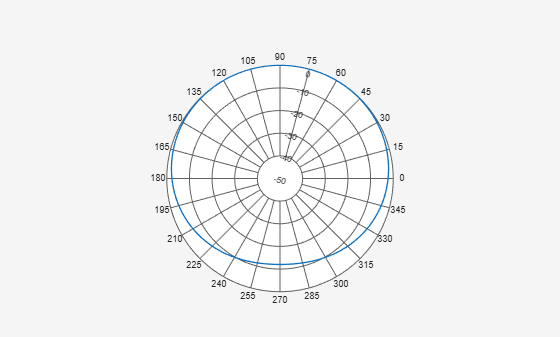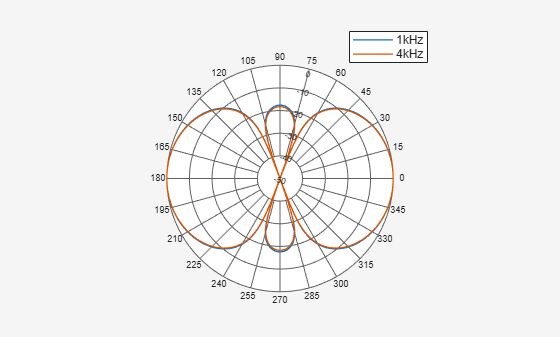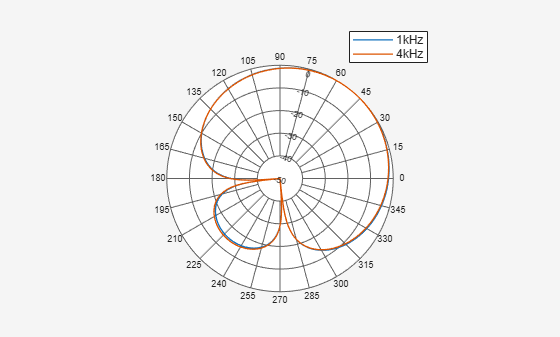# diffbfweights

Differential beamforming weights for ULA and UCA

## Syntax

``wts = diffbfweights(nelem,elemspacing)``
``wts = diffbfweights(nelem,elemspacing,ArrayGeometry='ULA')``
``wts = diffbfweights(nelem,rad,ArrayGeometry='UCA')``
``wts = diffbfweights(nelem,elemspacing,angc,ArrayGeometry=ag)``
``wts = diffbfweights(nelem,elemspacing,angc,respc,ArrayGeometry=ag)``
``wts = diffbfweights(___,SteerAngle=angs)``
``wts = diffbfweights(___,DiffuseNoiseCovariance=dncov)``
``wts = diffbfweights(___,DiagonalLoading=epsilon)``
``[wts,pos] = diffbfweights(___)``

## Description

example

````wts = diffbfweights(nelem,elemspacing)` computes differential beamforming weights that steer a uniform linear array (ULA) to its endfire direction. `nelem` is the number of elements in the array and `elemspacing` is the distance between array elements. Differential weights can achieve frequency invariant beam patterns and are often used in microphone arrays.```

example

````wts = diffbfweights(nelem,elemspacing,ArrayGeometry='ULA')` explicitly sets the array to a uniform linear array (ULA).```

example

````wts = diffbfweights(nelem,rad,ArrayGeometry='UCA')` computes the differential beamforming weights for a uniform circular array (UCA). `nelem` is the number of elements in the array and `rad` is the radius ot the array.```

example

````wts = diffbfweights(nelem,elemspacing,angc,ArrayGeometry=ag)` also specifies angles `angc` at which the array response is null. When `ag` equals 'ULA', `elemspacing` refers to the distance between array elements. When `ag` equals 'UCA', `elemspacing` refers to the array radius.```
````wts = diffbfweights(nelem,elemspacing,angc,respc,ArrayGeometry=ag)` also specifies the desired responses, `respc`, at the angles specified in `angc`. When `ag` equals 'ULA', `elemspacing` refers to the number of array elements, When `ag` equals 'UCA', `elemspacing` refers to the array radius.```
````wts = diffbfweights(___,SteerAngle=angs)` also specifies the array steering angle `angs`.```
````wts = diffbfweights(___,DiffuseNoiseCovariance=dncov)` also specifies the diffuse noise covariance matrix `dncov`.```
````wts = diffbfweights(___,DiagonalLoading=epsilon)` also specifies the diagonal loading factor `epsilon`.```
````[wts,pos] = diffbfweights(___)` also returns the element positions `pos`.```

## Examples

collapse all

Display the pattern of a four-element uniform linear array. The element spacing is 1/10 of the wavelength.

```N = 4; angp = -180:180; [w,pos] = diffbfweights(N,0.1,ArrayGeometry='ULA'); bp = arrayfactor(pos,angp,w); polarpattern(angp,mag2db(abs(bp)),'NormalizeData',true, ... 'MagnitudeLimMode','manual','MagnitudeLim',[-50 0])```Display the pattern of a six-element uniform circular array. The array radius is one wavelength.

```N = 6; angp = -180:180; [w,pos] = diffbfweights(N,1,ArrayGeometry='UCA'); bp = arrayfactor(pos,angp,w); polarpattern(angp,mag2db(abs(bp)),'NormalizeData',true, ... 'MagnitudeLimMode','manual','MagnitudeLim',[-50 0])```Create a cardioid pattern for a four-element uniform linear array. The main beam points to the endfire direction, 90 degrees azimuth, and the null is in the opposite direction. The element spacing is set to be 1/10 of the wavelength corresponding to the maximum frequency of interest.

```N = 4; angp = -180:180; [w,pos] = diffbfweights(4,0.1,-90); bp = arrayfactor(pos,angp,w); polarpattern(angp,mag2db(abs(bp)),'NormalizeData',true, ... 'MagnitudeLimMode','manual','MagnitudeLim',[-50 0])```Create a cardioid pattern with a four-element uniform linear array. Plot the pattern for both 1 kHz and 4 kHz. The main beam points to the broadside direction (0 degrees azimuth) and the null is at 70 degrees azimuth. The element spacing is set to be 1/10 of the wavelength corresponding to the maximum frequency of interest,

```N = 4; c = 343; fc = [1e3 4e3]; lambda = c./fc; d = 0.1*lambda(2); angp = -180:180; for m = 2:-1:1 [w,pos] = diffbfweights(N,d/lambda(m),70,SteerAngle=0); bp(:,m) = arrayfactor(pos,angp,w); end polarpattern(angp,mag2db(abs(bp)),'NormalizeData',true, ... 'MagnitudeLimMode','manual','MagnitudeLim',[-50 0]) legend('1kHz','4kHz')```Create a super-cardioid pattern with a 3-element uniform circular array. Plot the pattern for both 1 kHz and 4 kHz. The main beam points to 0 degrees azimuth and the null is at 135 degrees azimuth. The element spacing is set to be 1/10 of the wavelength corresponding to the maximum frequency of interest.

```N = 3; c = 343; fc = [1e3 4e3]; lambda = c./fc; d = 0.1*lambda(2); r = N*d/(2*pi); angp = -180:180; for m = 2:-1:1 [w,pos] = diffbfweights(N,r/lambda(m),135,'ArrayGeometry','UCA'); bp(:,m) = arrayfactor(pos,angp,w); end polarpattern(angp,mag2db(abs(bp)),'NormalizeData',true, ... 'MagnitudeLimMode','manual','MagnitudeLim',[-50 0]) legend('1kHz','4kHz')```Create a super-cardioid pattern with a four-element uniform circular array. Plot the pattern for both 1 kHz and 4 kHz. The main beam points to 50 degrees azimuth and the null is at 185 degrees azimuth. The radius is set to be 1/10 of the wavelength corresponding to the maximum frequency of interest.

```N = 4; c = 343; fc = [1e3 4e3]; lambda = c./fc; r = 0.1*lambda(2); angp = -180:180; for m = 2:-1:1 [w,pos] = diffbfweights(N,r/lambda(m),185, ... SteerAngle=50,ArrayGeometry='UCA'); bp(:,m) = arrayfactor(pos,angp,w); end polarpattern(angp,mag2db(abs(bp)),'NormalizeData',true, ... 'MagnitudeLimMode','manual','MagnitudeLim',[-50 0]) legend('1kHz','4kHz')```## Input Arguments

collapse all

Number of array element, specified as a positive integer.

Data Types: `double`

Element spacing of a ULA array, specified as a positive scalar. Units are in wavelength.

#### Dependencies

To enable this argument, set the `ArrayGeometry` property to `'ULA'`.

Data Types: `double`

Radius of UCA, specified as a positive scalar. Units are in wavelength.

#### Dependencies

To enable this argument, set the `ArrayGeometry` property to `'UCA'`.

Data Types: `double`

Array geometry, specified as `'ULA'` or `'UCA'`. `'ULA'` represent a uniform linear array and `'UCA'` represents a uniform circular array. Use this argument to set the value of the `ArrayGeometry` property.

Data Types: `double`

Angular directions of null responses measured from broadside, specified as a real-valued P-element row vector. Angles must lie in the interval [–90,90].

Data Types: `double`

Response at the null angles defined by `angc`, specified as a real-valued P-element row vector. The number of entries in `respc` must be equal to the number of entries in `angc`.

Data Types: `double`

Steering vector direction of the array, specified as a scalar in the interval [–90, 90]. The angle is measured from broadside. This argument sets the value of the `SteerAngle` property. Units are in degrees.

Data Types: `double`

Diffuse covariance noise matrix, specified as an N-by-N matrix. Diffuse noise describes the noise presented at different directions. The default value is an identity matrix, indicating the diffuse noise is spatially white. This argument sets the value of the `DiffuseNoiseCovariance` property.

Data Types: `double`

Diagonal loading factor, specified as as a nonnegative scalar. The diagonal loading is used to form a more robust estimate of the covariance. Units are dimensionless. This argument sets the value of the `DiagonalLoading` property.

Data Types: `double`

## Output Arguments

collapse all

Array weights, returned as an N-element complex-valued vector. Values are dimensionless.

Array element positions, returned as a 3-by-N real-valued matrix where N is the number of array elements. Each column of `pos` represents the [x;y;z] coordinates of the corresponding element. For a ULA, the returned array is along y-axis, located at (0:N-1)*D. For a UCA, the returned array is in the x-y-plane, with its first element on zero degrees azimuth. Units are in wavelengths.

 Benesty, Jacob, et al. Fundamentals of Differential Beamforming. Springer Singapore, 2016. DOI.org (Crossref), https://doi.org/10.1007/978-981-10-1046-0.

 Benesty, Jacob, and J. Chen. Study and Design of Differential Microphone Arrays. Springer, 2013.

 Jingdong Chen, Jacob Benesty, and Chao Pan, "On the design and implementation of linear differential microphone arrays", The Journal of the Acoustical Society of America, Vol 136, pp 3097, 2014.

 Jilu Jin, Gongping Huang, Xuehan Wang, Jingdong Chen, Jacob Benesty, and Israel Cohen, "Steering Study of Linear Differential Microphone Arrays", IEEE/ACM Transactions on Audio, Speech, and Language Processing, Vol 29, pp 158, 2020

 Jacob Benesty, Jingdong Chen, and Israel Cohen, Design of Circular Differential Microphone Arrays, Springer 2015.

 Gongping Huang, Israel Cohen, Jingdong Chen, and Jacob Benesty, "Continuously steerable differential beamformers with null constraints for circular microphone arrays", The Journal of the Acoustical Society of America, Vol 148, pp 1248, 2020.